# Duncan Lardeau Juvenile Rainbow Trout Abundance 2021

The suggested citation for this analytic report is:

Thorley, J.L. and Amies-Galonski, E. (2021) Duncan Lardeau Juvenile Rainbow Trout Abundance 2021. A Poisson Consulting Analysis Appendix. URL: https://www.poissonconsulting.ca/f/1275560691.

## Background

Rainbow Trout rear in the Lardeau and Lower Duncan rivers. Since 2006 (with the exception of 2015) annual spring snorkel surveys have been conducted to estimate the abundance and distribution of age-1 Rainbow Trout. From 2006 to 2010 the surveys were conducted at fixed index sites. Since 2011 fish observations have been mapped to the river based on their spatial coordinates as recorded by GPS.

The primary aims of the current analyses were to:

• Estimate the spring abundance of age-1 fish by year.
• Estimate the egg deposition.
• Estimate the stock-recruitment relationship between the egg deposition and the abundance of age-1 recruits the following spring.
• Estimate the survival from age-1 to age-2.
• Estimate the expected number of spawners without in river and/or in lake variation.

## Methods

### Data Preparation

The data were provided by the Ministry of Forests, Lands and Natural Resource Operations (MFLNRO). The historical and current snorkel count data were manipulated using R version 4.1.0 and organised in an SQLite database.

### Statistical Analysis

Model parameters were estimated using Bayesian methods. The estimates were produced using JAGS and STAN . For additional information on Bayesian estimation the reader is referred to , respectively.

Unless stated otherwise, the Bayesian analyses used weakly informative normal and half-normal prior distributions . The posterior distributions were estimated from 1500 Markov Chain Monte Carlo (MCMC) samples thinned from the second halves of 3 chains . Model convergence was confirmed by ensuring that the potential scale reduction factor $$\hat{R} \leq 1.05$$ and the effective sample size $$\textrm{ESS} \geq 150$$ for each of the monitored parameters .

The parameters are summarised in terms of the point estimate, lower and upper 95% credible limits (CLs) and the surprisal s-value . The estimate is the median (50th percentile) of the MCMC samples while the 95% CLs are the 2.5th and 97.5th percentiles. The s-value can be considered a test of directionality. More specifically it indicates how surprising (in bits) it would be to discover that the true value of the parameter is in the opposite direction to the estimate. An s-value of 4.3 bits, which is equivalent to a p-value of 0.05, indicates that the surprise would be equivalent to throwing 4.3 heads in a row.

The results are displayed graphically by plotting the modeled relationships between particular variables and the response(s) with the remaining variables held constant. In general, continuous and discrete fixed variables are held constant at their mean and first level values, respectively, while random variables are held constant at their typical values (expected values of the underlying hyperdistributions) . When informative the influence of particular variables is expressed in terms of the effect size (i.e., percent or n-fold change in the response variable) with 95% credible intervals .

The analyses were implemented using R version 4.1.0 and the mbr family of packages.

### Model Descriptions

#### Length Correction

The annual bias (inaccuracy) and error (imprecision) in observer’s fish length estimates when spotlighting (standing) and snorkeling were quantified from the divergence of their length distribution from the length distribution for all observers (including measured fish) in that year. More specifically, the length correction that minimised the Jensen-Shannon divergence (Lin 1991) between the two distributions provided a measure of the inaccuracy while the minimum divergence (the Jensen-Shannon divergence was calculated with log to base 2 which means it lies between 0 and 1) provided a measure of the imprecision.

After correcting the fish lengths, age-1 individuals were assumed to be those with a fork length $$\leq$$ 100 mm.

#### Abundance

The abundance was estimated from the count data using an overdispersed Poisson model . The annual abundance estimates represent the total number of fish in the study area.

Key assumptions of the abundance model include:

• The lineal fish density varies with year, useable width and river kilometer as a polynomial, and randomly with site.
• The observer efficiency at marking sites varies by study design (GPS versus Index).
• The observer efficiency also varies by visit type (marking versus count) within study design and randomly by snorkeller.
• The expected count at a site is the expected lineal density multiplied by the site length, the observer efficiency and the proportion of the site surveyed.
• The residual variation in the actual count is gamma-Poisson distributed.

#### Condition

The condition of fish with a fork length $$\geq$$ 500 mm was estimated via an analysis of mass-length relations .

More specifically the model was based on the allometric relationship

$W = \alpha_c L^{\beta_c}$

where $$W$$ is the weight (mass), $$\alpha_c$$ is the coefficent, $$\beta_c$$ is the exponent and $$L$$ is the length.

To improve chain mixing the relation was log-transformed, i.e.,

$\log(W) = \log(\alpha_c) + \beta_c \log(L).$

Key assumptions of the condition model include:

• $$\alpha_c$$ can vary randomly by year.
• The residual variation in weight is log-normally distributed.

#### Fecundity

The fecundity of females with a fork length $$\geq$$ 500 mm was estimated via an analysis of fecundity-mass relations.

More specifically the model was based on the allometric relationship

$F = \alpha_f W^{\beta_f}$

where $$F$$ is the fecundity, $$\alpha_f$$ is the coefficent, $$\beta_f$$ is the exponent and $$W$$ is the weight.

To improve chain mixing the relation was log-transformed.

Key assumptions of the fecundity model include:

• The residual variation in fecundity is log-normally distributed.

#### Spawner Size

The average length of the spawners in each year (for years for which it was unavailable) was estimated from the mean weight of Rainbow Trout in the Kootenay Lake Rainbow Trout Mailout Survey (KLRT) using a linear regression. This approach was suggested by Rob Bison.

#### Egg Deposition

The egg deposition in each year was estimated by

1. converting the average length of spawners to the average weight using the condition relationship for a typical year
2. adjusting the average weight by the annual condition effect (interpolating where unavailable)
3. converting the average weight to the average fecundity using the fecundity relationship
4. multiplying the average fecundity by the AUC based estimate of the number of females (assuming a sex ratio of 1:1)

#### Stock-Recruitment

The relationship between the number of eggs ($$E$$) and the abundance of age-1 individuals the following spring ($$R$$) was estimated using a Beverton-Holt stock-recruitment model :

$R = \frac{\alpha_s \cdot E}{1 + \beta_s \cdot E} \quad,$

where $$\alpha_s$$ is the maximum number of recruits per egg (egg survival), and $$\beta_s$$ is the density dependence.

Key assumptions of the stock-recruitment model include:

• The residual variation in the number of recruits is log-normally distributed with the standard deviation scaling with the uncertainty in the number of recruits.

The age-1 carrying capacity ($$K$$) is given by:

$K = \frac{\alpha_s}{\beta_s} \quad.$

and the $$E_{K/2}$$ Limit Reference Point , which corresponds to the stock (number of eggs) that produce 50% of the maximum recruitment ($$K$$), by $E_{K/2} = \frac{1}{\beta_s}$

The LRP was also converted into a number of spawners in a typical year (assuming 6,000 eggs per spawner and a sex ratio of 1:1).

#### Age-1 to Age-2 Survival

The relationship between the number of age-1 individuals and the number of age-2 individuals the following year was estimated using a linear regression through the origin where the slope was constrained to lie between 0 and 1 by a logistic transformation.

Key assumptions of the survival rate model include:

• The residual variation in the number of age-2 individuals is log-normally distributed.

#### Reproductive Rate

The maximum reproductive rate (the number of spawners per spawner at low density) not accounting for fishing mortality was calculated by multiplying $$\beta_s$$ (number of recruits per egg at low density) from the stock-recruitment relationship by the inlake survival by the estimated eggs per spawner in each year (assuming a sex ratio of 1:1). The inlake survival from age-1 to spawning was calculated by dividing the subsequent number of spawners by the number of recruits assuming that equal numbers of fish spawn at age 5, 6 and 7.

#### Expected Spawners

The expected spawners without in river and/or in lake variation was calculated from the stock-recruitment relationship and assuming an age-1 to spawner survival of 0.68%.

## Results

### Model Templates

#### Abundance

  data {

int<lower=0> nMarked;
int<lower=0> Marked[nMarked];
int<lower=0> Resighted[nMarked];
int<lower=0> IndexMarked[nMarked];

int<lower=0> nObs;
int Marking[nObs];
int Index[nObs];
int<lower=0> nSwimmer;
int<lower=0> Swimmer[nObs];
int<lower=0> nYear;
int<lower=0> Year[nObs];
real Rkm[nObs];
int<lower=0> nSite;
int<lower=0> Site[nObs];

real SiteLength[nObs];
real SurveyProportion[nObs];

int Count[nObs];
}
parameters {
real bEfficiency;
real bEfficiencyIndex;

real bDensity;
real<lower=0> sDensityYear;
vector[nYear] bDensityYear;

vector bDensityRkm;
real<lower=0> sDensitySite;
vector[nSite] bDensitySite;

real bEfficiencyMarking;
real bEfficiencyMarkingIndex;

real<lower=0,upper=5> sEfficiencySwimmer;
vector[nSwimmer] bEfficiencySwimmer;

real<lower=0> sDispersion;
}
model {

vector[nObs] eDensity;
vector[nObs] eEfficiency;
vector[nObs] eAbundance;
vector[nObs] eCount;

sDispersion ~ gamma(0.01, 0.01);

bDensity ~ normal(0, 2);
bDensityRkm ~ normal(0, 2);
sDensitySite ~ uniform(0, 5);
bDensitySite ~ normal(0, sDensitySite);
sDensityYear ~ uniform(0, 5);
bDensityYear ~ normal(0, sDensityYear);

bEfficiency ~ normal(0, 5);
bEfficiencyIndex ~ normal(0, 5);
bEfficiencyMarking ~ normal(0, 5);
bEfficiencyMarkingIndex ~ normal(0, 5);
sEfficiencySwimmer ~ uniform(0, 5);
bEfficiencySwimmer ~ normal(0, sEfficiencySwimmer);

for (i in 1:nMarked) {
target += binomial_lpmf(Resighted[i] | Marked[i],
inv_logit(
bEfficiency +
bEfficiencyIndex * IndexMarked[i] +
bEfficiencyMarking +
bEfficiencyMarkingIndex * IndexMarked[i]
));
}

for (i in 1:nObs) {
eDensity[i] = exp(bDensity +
bDensityRkm * Rkm[i] +
bDensityRkm * pow(Rkm[i], 2.0) +
bDensityRkm * pow(Rkm[i], 3.0) +
bDensityRkm * pow(Rkm[i], 4.0) +
bDensitySite[Site[i]] +
bDensityYear[Year[i]]);

eEfficiency[i] = inv_logit(
bEfficiency +
bEfficiencyIndex * Index[i] +
bEfficiencyMarking * Marking[i] +
bEfficiencyMarkingIndex * Index[i] * Marking[i] +
bEfficiencySwimmer[Swimmer[i]]);

eAbundance[i] = eDensity[i] * SiteLength[i];

eCount[i] = eAbundance[i] * eEfficiency[i] * SurveyProportion[i];
}

target += neg_binomial_2_lpmf(Count | eCount, sDispersion);
}

Block 1. Abundance model description.

#### Condition

 data {
int nYear;
int nObs;

vector[nObs] Length;
vector[nObs] Weight;
int Year[nObs];

parameters {
real bWeight;
real bWeightLength;
real sWeightYear;

vector[nYear] bWeightYear;
real sWeight;

model {

vector[nObs] eWeight;

bWeight ~ normal(-10, 5);
bWeightLength ~ normal(3, 2);

sWeightYear ~ normal(-2, 5);

for (i in 1:nYear) {
bWeightYear[i] ~ normal(0, exp(sWeightYear));
}

sWeight ~ normal(-2, 5);
for(i in 1:nObs) {
eWeight[i] = bWeight + bWeightLength * log(Length[i]) + bWeightYear[Year[i]];
Weight[i] ~ lognormal(eWeight[i], exp(sWeight));
}

Block 2.

#### Fecundity

 data {
int nObs;

vector[nObs] Weight;
vector[nObs] Fecundity;

parameters {
real bFecundity;
real bFecundityWeight;
real sFecundity;

model {

vector[nObs] eFecundity;

bFecundity ~ uniform(0, 5);
bFecundityWeight ~ uniform(0, 2);

sFecundity ~ uniform(0, 1);
for(i in 1:nObs) {
eFecundity[i] = log(bFecundity) + bFecundityWeight * log(Weight[i]);
Fecundity[i] ~ lognormal(eFecundity[i], sFecundity);
}

Block 3.

#### Stock-Recruitment

model {
a ~ dunif(0, 1)
b ~ dunif(0, 0.1)
sScaling ~ dunif(0, 5)

eRecruits <- a * Stock / (1 + Stock * b)

for(i in 1:nObs) {
esRecruits[i] <- SDLogRecruits[i] * sScaling
Recruits[i] ~ dlnorm(log(eRecruits[i]), esRecruits[i]^-2)
}

Block 4. Stock-Recruitment model description.

#### Age-1 to Age-2 Survival

model {
bSurvival ~ dnorm(0, 2^-2)
sRecruits ~ dnorm(0, 2^-2) T(0,)

for(i in 1:nObs) {
logit(eSurvival[i]) <- bSurvival
eRecruits[i] <- Stock[i] * eSurvival[i]
Recruits[i] ~ dlnorm(log(eRecruits[i]), sRecruits^-2)
}

Block 5. In-river survival model description.

### Tables

#### Abundance

Table 1. Parameter descriptions.

Parameter Description
bDensity Intercept for log(eDensity)
bDensityRkm[i] ith-order polynomial coefficients of effect of river kilometer on bDensity
bDensitySite[i] Effect of ith Site on bDensity
bDensityYear[i] Effect of ith Year on bDensity
bEfficiency Intercept of logit(eEfficiency)
bEfficiencyIndex Effect of Index on bEfficiency
bEfficiencyMarking Effect of Marking on bEfficiency
bEfficiencyMarkingIndex Effect of Marking and Index on bEfficiency
bEfficiencySwimmer[i] Effect of ith Swimmer on bEfficiency
eAbundance[i] Expected abundance of fish at site of ith visit
eCount[i] Expected total number of fish at site of ith visit
eDensity[i] Expected lineal density of fish at site of ith visit
eEfficiency[i] Expected observer efficiency on ith visit
Index Whether the ith visit was to an index site
Marking[i] Whether the ith visit was to a site with marked fish
Rkm[i] River kilometer of ith visit
sDensitySite SD of bDensitySite
sDispersion Overdispersion of Count[i]
sEfficiencySwimmer SD of bEfficiencySwimmer
Site[i] Site of ith visit
SiteLength[i] Length of site of ith visit
SurveyProportion[i] Proportion of site surveyed on ith visit
Swimmer[i] Snorkeler on ith site visit
Year[i] Year of ith site visit
##### Age-1

Table 2. Model coefficients.

term estimate lower upper svalue
bDensity -1.2098091 -1.6747048 -0.6972998 10.551708
bDensityRkm -0.1927617 -0.3440534 -0.0359800 6.303781
bDensityRkm 0.5900044 0.3786136 0.7952371 10.551708
bDensityRkm -0.1132496 -0.1922307 -0.0411864 8.966746
bDensityRkm -0.2771688 -0.3516006 -0.2055780 10.551708
bEfficiency -1.8013903 -2.0749370 -1.5160279 10.551708
bEfficiencyIndex 0.3369932 -0.2483043 0.9882405 1.840902
bEfficiencyMarking 0.4952944 0.2727450 0.7038776 10.551708
bEfficiencyMarkingIndex 0.9203477 0.2690139 1.5397472 7.092277
sDensitySite 0.6610888 0.5943993 0.7335773 10.551708
sDensityYear 0.6717474 0.4612568 1.0363068 10.551708
sDispersion 1.3056903 1.1739344 1.4479836 10.551708
sEfficiencySwimmer 0.4678608 0.2768795 0.8591817 10.551708

Table 3. Model summary.

n K nchains niters nthin ess rhat converged
3508 13 3 500 5 759 1.007 TRUE

Table 4. Model posterior predictive checks.

moment observed median lower upper svalue
zeros 0.0000000 0.0000000 0.0000000 0.0000000 0.00000
mean -0.3005236 -0.3681359 -0.4018284 -0.3352433 10.55171
variance 0.6007891 0.8558667 0.8133539 0.8987680 10.55171
skewness 0.1858572 0.5375591 0.4573260 0.6230798 10.55171
kurtosis -0.5426561 -0.1995929 -0.3723576 0.0247367 10.55171
##### Age-2

Table 5. Model coefficients.

term estimate lower upper svalue
bDensity -2.0842028 -2.5926068 -1.5915817 10.5517083
bDensityRkm -0.2128592 -0.4130666 -0.0334140 5.3422549
bDensityRkm 0.0336427 -0.2243816 0.2757409 0.3265008
bDensityRkm 0.0425520 -0.0489595 0.1355835 1.5601864
bDensityRkm -0.0945494 -0.1781345 -0.0060137 5.0598552
bEfficiency -2.0329898 -2.4692558 -1.5949384 10.5517083
bEfficiencyIndex 0.5349712 -0.1192730 1.2363483 3.2029801
bEfficiencyMarking 0.7176875 0.3992786 1.0513500 10.5517083
bEfficiencyMarkingIndex 2.1668745 1.1393489 3.2477195 10.5517083
sDensitySite 0.7740179 0.6865336 0.8684278 10.5517083
sDensityYear 0.5460727 0.3690789 0.8834438 10.5517083
sDispersion 1.1716236 1.0111061 1.3885311 10.5517083
sEfficiencySwimmer 0.3873169 0.2325397 0.6511071 10.5517083

Table 6. Model summary.

n K nchains niters nthin ess rhat converged
3508 13 3 500 5 768 1.009 TRUE

Table 7. Model posterior predictive checks.

moment observed median lower upper svalue
zeros 0.0000000 0.0000000 0.0000000 0.0000000 0.0009615
mean -0.3093311 -0.3362331 -0.3670306 -0.3078566 3.7835239
variance 0.5801792 0.7274513 0.6770241 0.7744862 10.5517083
skewness 0.7303687 0.8791963 0.7842122 0.9798257 10.5517083
kurtosis -0.1378032 0.3223071 0.0477461 0.6584863 10.5517083

#### Condition

Table 8. Parameter descriptions.

Parameter Description
bWeight Intercept of log(eWeight)
bWeightLength Intercept of effect of log(Length) on bWeight
bWeightYear[i] Effect of ith Year on bWeight
eWeight[i] Expected Weight of ith fish
Length[i] Fork length of ith fish
sWeight Log standard deviation of residual variation in log(Weight)
sWeightYear Log standard deviation of bWeightYear
Weight[i] Recorded weight of ith fish
Year[i] Year ith fish was captured

Table 9. Model coefficients.

term estimate lower upper svalue
bWeight -8.957515 -9.577279 -8.327107 10.55171
bWeightLength 2.636533 2.540749 2.729346 10.55171
sWeight -1.412853 -1.451705 -1.369734 10.55171
sWeightYear -1.593725 -1.897886 -1.251610 10.55171

Table 10. Model summary.

n K nchains niters nthin ess rhat converged
1185 4 3 500 2 304 1.005 TRUE

Table 11. Model posterior predictive checks.

moment observed median lower upper svalue
zeros 0.0000000 0.0000000 0.0000000 0.0000000 0.0000000
mean 0.0007358 -0.0017763 -0.0831461 0.0801327 0.0851219
variance 1.9582756 2.0009886 1.8506364 2.1637770 0.7235718
skewness -11.6566714 -0.0012152 -0.1455530 0.1380060 10.5517083
kurtosis 287.3216012 -0.0043032 -0.2486362 0.3319438 10.5517083

Table 12. Model sensitivity.

n K nchains niters rhat_1 rhat_2 rhat_all converged
1185 4 3 500 1.005 1.008 1.004 TRUE

#### Fecundity

Table 13. Parameter descriptions.

Parameter Description
bFecundity Intercept of eFecundity
bFecundityWeight Effect of log(Weight) on log(bFecundity)
eFecundity[i] Expected Fecundity of ith fish
Fecundity[i] Fecundity of ith fish (eggs)
sFecundity SD of residual variation in log(Fecundity)
Weight[i] Weight of ith fish (mm)

Table 14. Model coefficients.

term estimate lower upper svalue
bFecundity 3.8649273 1.4275232 4.9500189 10.55171
bFecundityWeight 0.8610988 0.8317531 0.9739412 10.55171
sFecundity 0.1273735 0.0932693 0.1865299 10.55171

Table 15. Model summary.

n K nchains niters nthin ess rhat converged
22 3 3 500 2 262 1.016 TRUE

Table 16. Model posterior predictive checks.

moment observed median lower upper svalue
zeros 0.0000000 0.0000000 0.0000000 0.0000000 0.0000000
mean 0.0145273 0.0117784 -0.5468271 0.5663281 0.0077098
variance 1.8242731 1.9354759 0.9661501 3.4151509 0.2536457
skewness -2.1200692 0.0162370 -0.9750692 0.9409571 10.5517083
kurtosis 6.5274329 -0.4055770 -1.2334752 1.9683889 8.9667458

Table 17. Model sensitivity.

n K nchains niters rhat_1 rhat_2 rhat_all converged
22 3 3 500 1.016 1.006 1.01 TRUE

#### Stock-Recruitment

Table 18. Parameter descriptions.

Parameter Description
a Recruits per Stock at low density
b Density-dependence
eRecruits[i] Expected number of recruits from ith spawn year
esRecruits[i] Expected SD of residual variation in Recruits
Recruits[i] Number of recruits from ith spawn year
SDLogRecruits[i] Standard deviation of uncertainty in log(Recruits[i])
sScaling Scaling term for SD of residual variation in log(eRecruits)
Stock[i] Number of egg in ith spawn year
##### Age-1

Table 19. Model coefficients.

term estimate lower upper svalue
a 0.4838583 0.2211006 0.9626915 10.55171
b 0.0000046 0.0000015 0.0000116 10.55171
sScaling 2.4329909 1.6894008 3.9092801 10.55171

Table 20. Model summary.

n K nchains niters nthin ess rhat converged
15 3 3 500 100 1264 1.001 TRUE

Table 21. Estimated carry capacity (with 95% CRIs).

estimate lower upper
104000 70400 165000

Table 22. Model posterior predictive checks.

moment observed median lower upper svalue
zeros 0.0000000 0.0000000 0.0000000 0.0000000 0.0000000
mean -0.0303758 0.0317910 -0.6874105 0.7585917 0.1690842
variance 9.9527274 1.9560945 0.8217253 3.7740285 10.5517083
skewness 0.0010579 -0.0071256 -1.0580164 1.0567720 0.0271665
kurtosis -0.7404681 -0.5417368 -1.3705987 1.6298662 0.3805315

Table 23. Estimated reference points (with 80% CRIs).

Metric estimate lower upper
eggs 218000.00000 106000.00000 471000
spawners 72.66667 35.33333 157

Table 24. Model sensitivity.

n K nchains niters rhat_1 rhat_2 rhat_all converged
15 3 3 500 1.001 1.003 1.001 TRUE

#### Age-1 to Age-2 Survival

Table 25. Parameter descriptions.

Parameter Description
bSurvival logit(eSurvival)
eSurvival[i] Expected annual survival for ith spawn year
Recruits[i] Number of age-2 juveniles from ith spawn year
sRecruits SD of residual variation in Recruits
Stock[i] Number of age-1 juveniles from ith spawn year
##### Age-2

Table 26. Model coefficients.

term estimate lower upper svalue
bSurvival -0.8812839 -1.2203869 -0.506210 7.092277
sRecruits 0.4343028 0.2979112 0.713525 10.551708

Table 27. Model summary.

n K nchains niters nthin ess rhat converged
13 2 3 500 1 555 1.006 TRUE

Table 28. Model posterior predictive checks.

moment observed median lower upper svalue
zeros 0.0000000 0.0000000 0.0000000 0.0000000 0.0000000
mean -0.0467403 -0.0029952 -0.8161317 0.7575169 0.1202107
variance 1.7252479 1.8310607 0.7654493 3.7019920 0.1821109
skewness -0.1959049 0.0110819 -1.0891284 1.0667994 0.5446810
kurtosis -1.2697298 -0.6210505 -1.3920507 1.4292329 2.8169986

Table 29. Model sensitivity.

n K nchains niters rhat_1 rhat_2 rhat_all converged
13 2 3 500 1.006 1.003 1.004 TRUE

### Figures

#### Abundance

##### Age-1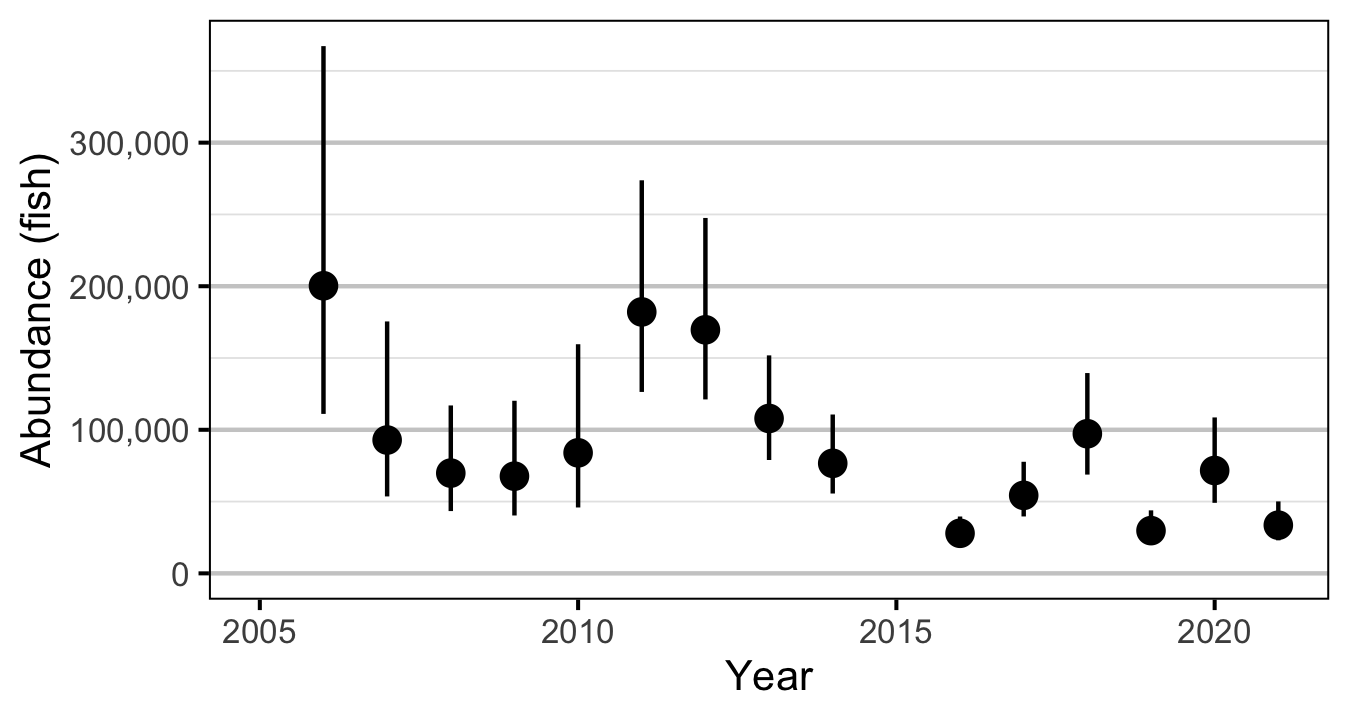Figure 3. Predicted abundance of age-1 Rainbow Trout in the Duncan and Lardeau Rivers by year (with 95% CRIs).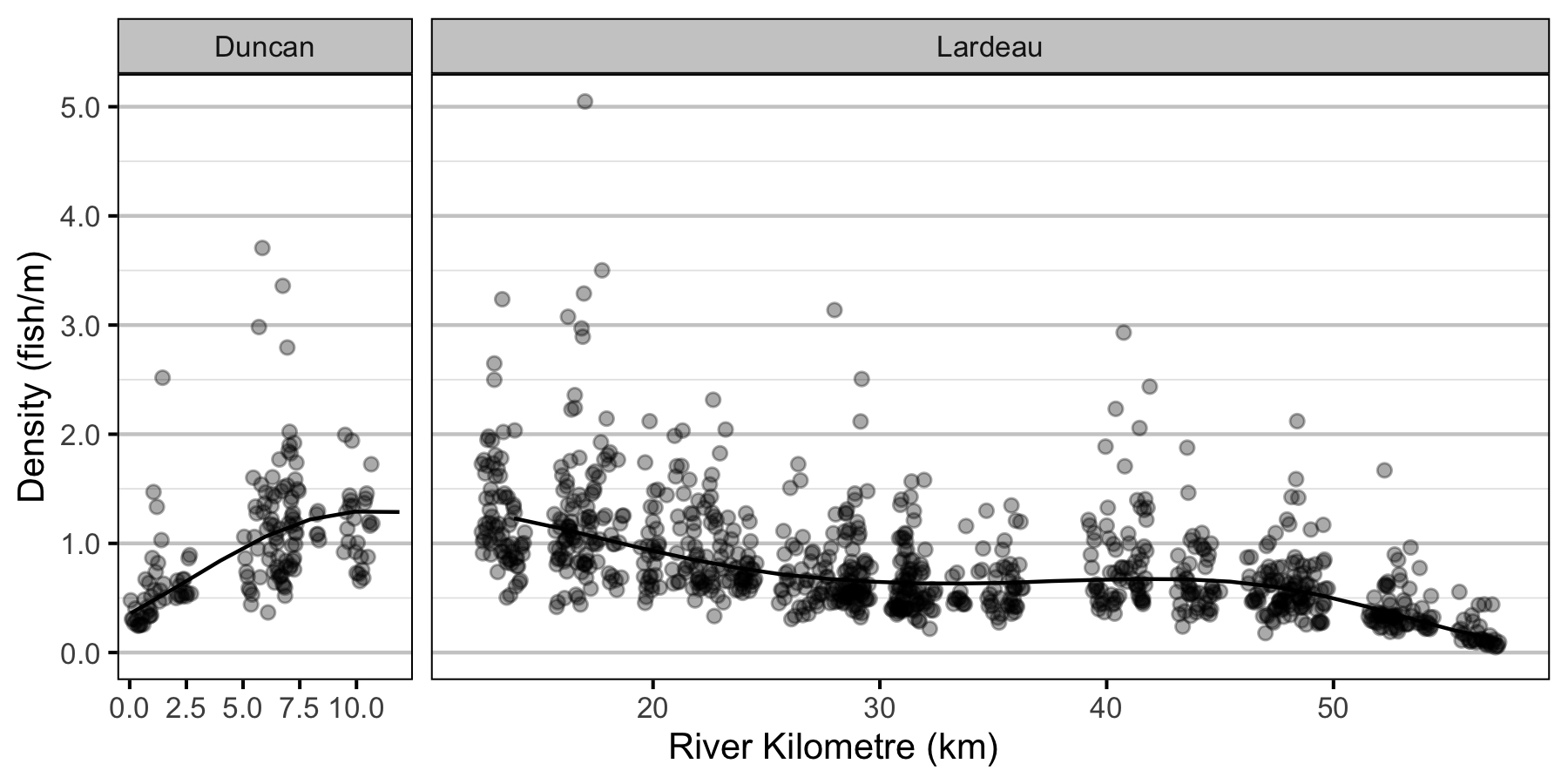Figure 4. Predicted lineal density of age-1 Rainbow Trout in 2010 by river kilometre (with 95% CRIs).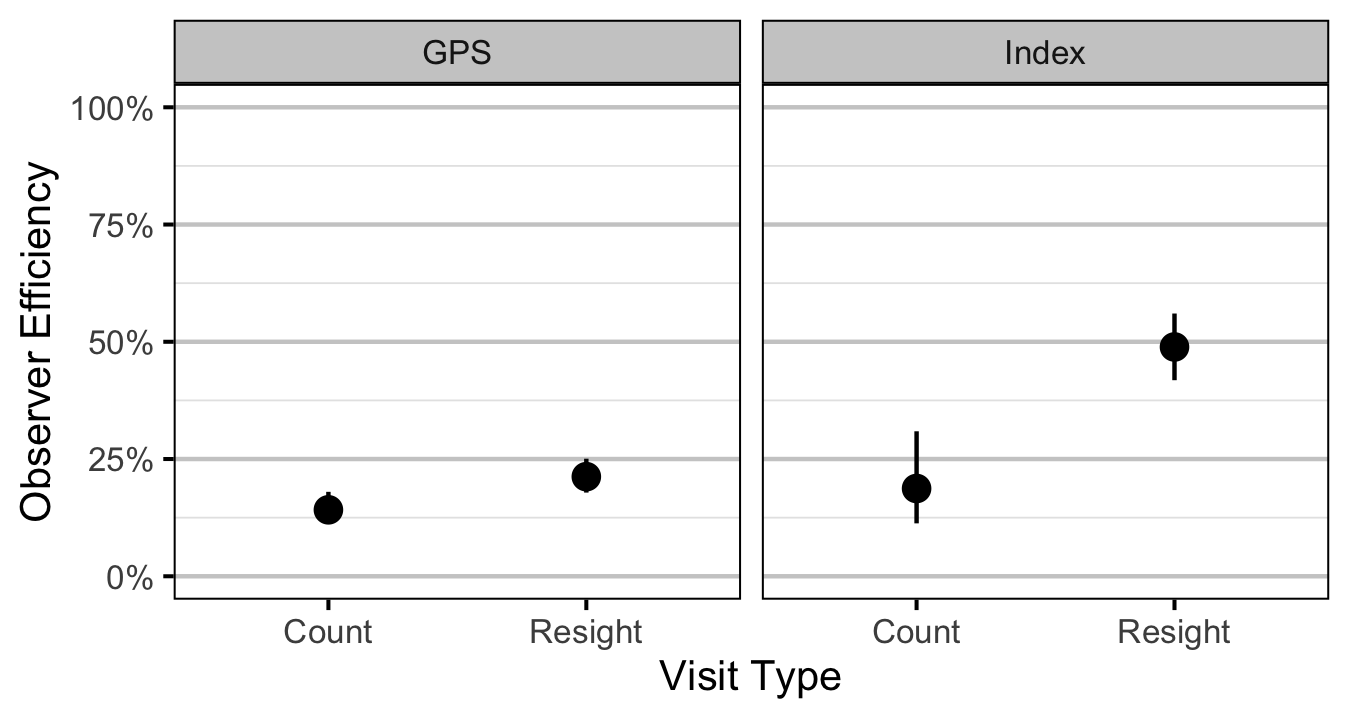Figure 5. Predicted observer efficiency for age-1 Rainbow Trout by visit type and study design (with 95% CRIs).
##### Age-2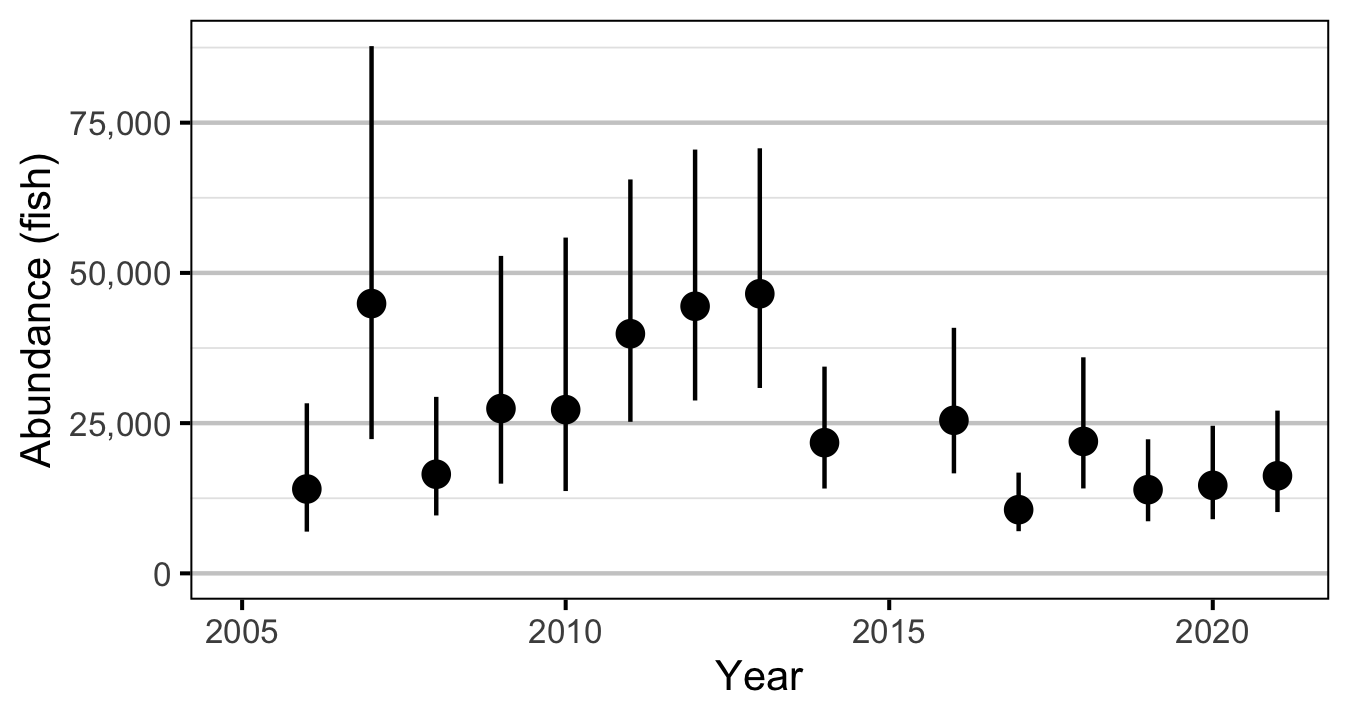Figure 6. Predicted abundance of age-2 Rainbow Trout in the Duncan and Lardeau Rivers by year (with 95% CRIs).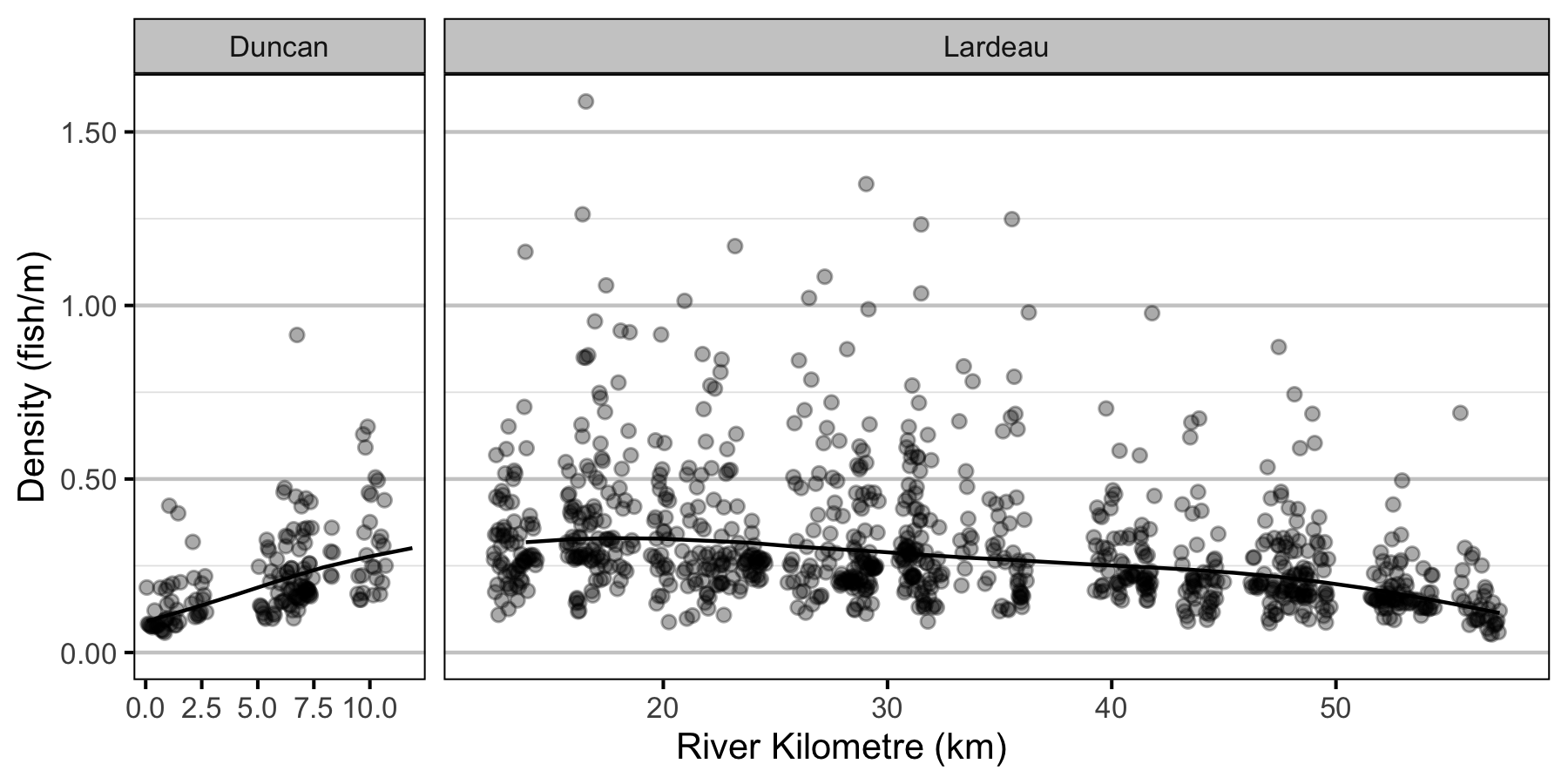Figure 7. Predicted lineal density of age-2 Rainbow Trout in 2010 by river kilometre (with 95% CRIs).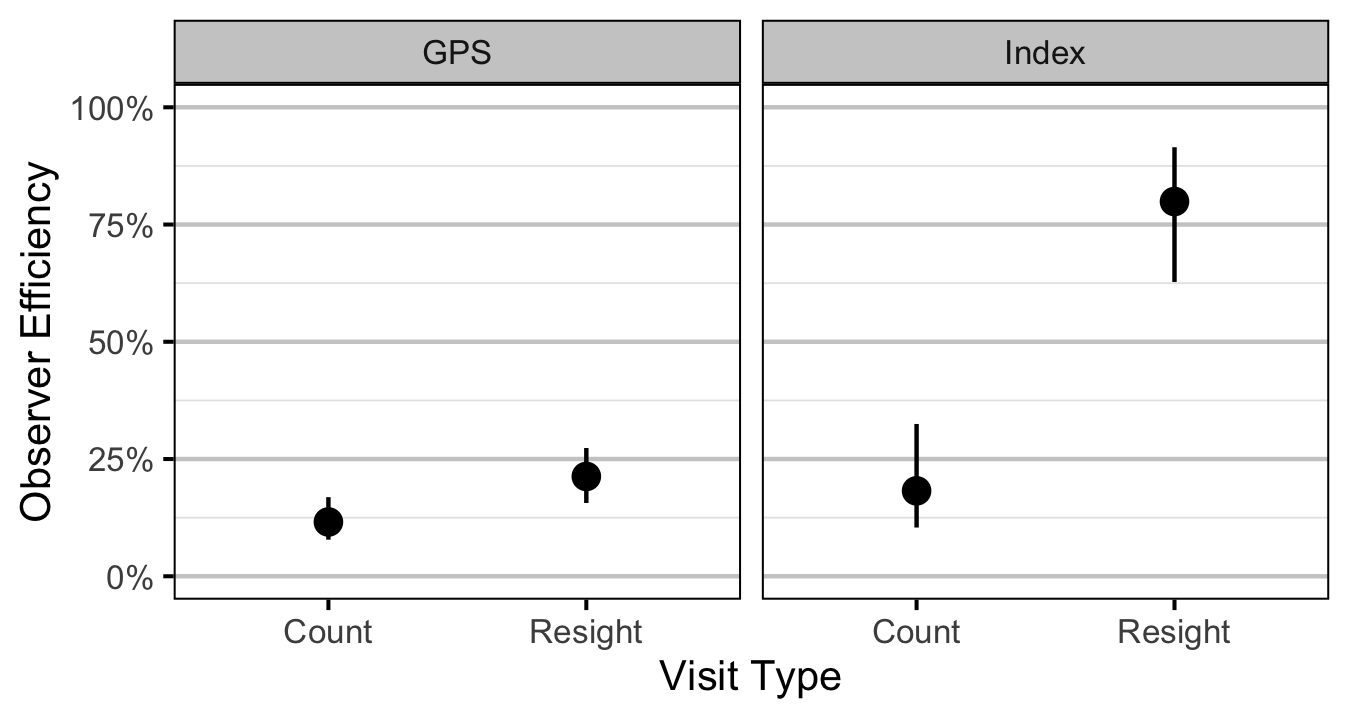Figure 8. Predicted observer efficiency for age-2 Rainbow Trout by visit type and study design (with 95% CRIs).

#### Condition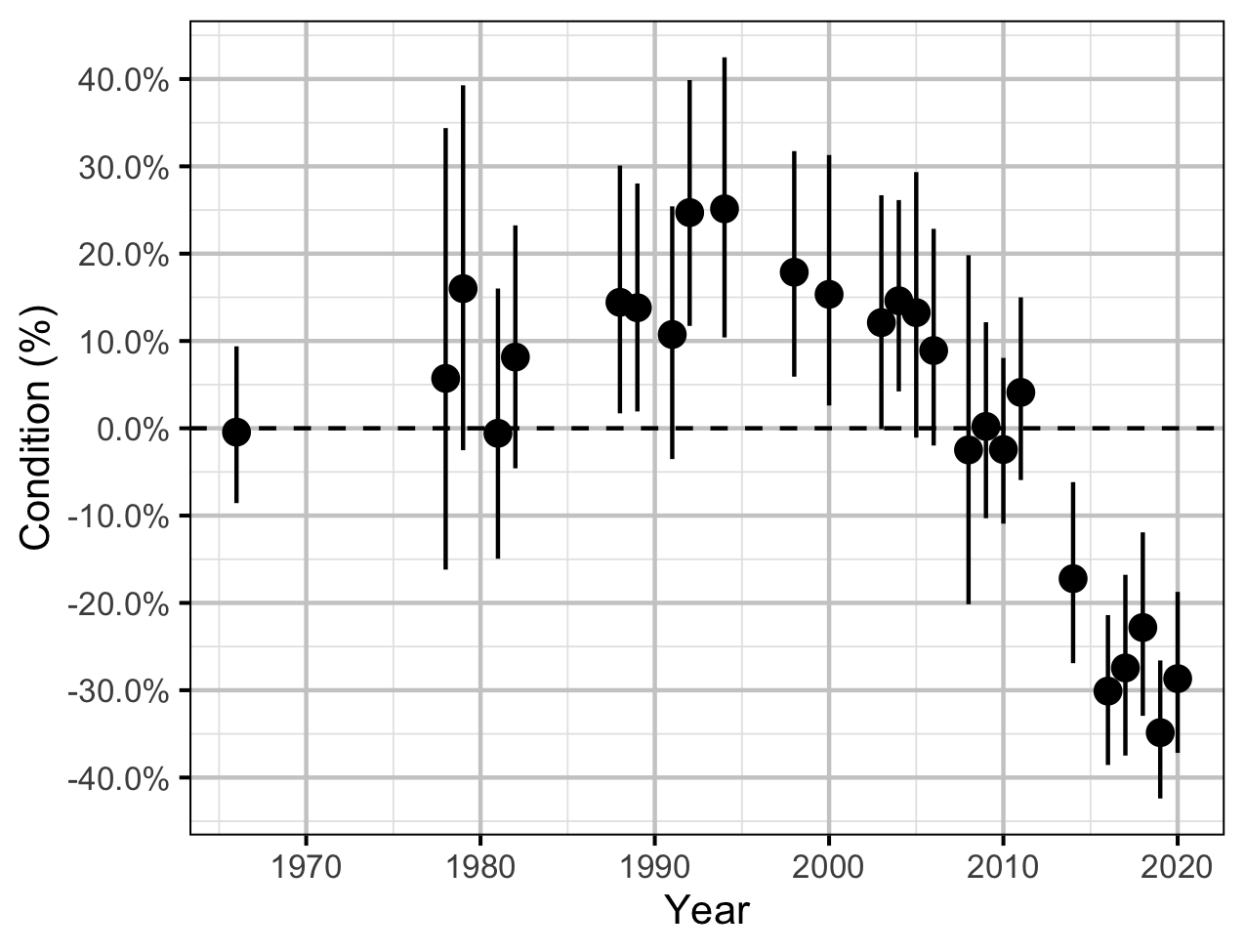Figure 9. The percent change in the body condition for an average length fish relative to a typical year by year (with 95% CRIs).

#### Spawner Size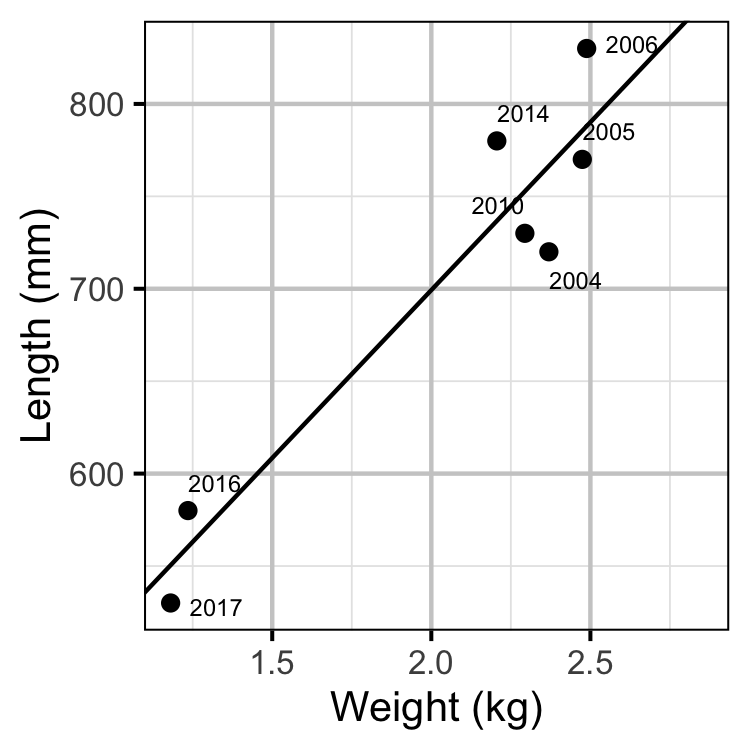Figure 11. The mean length of spawning Rainbow Trout by the mean weight of Rainbow Trout in the KLRT.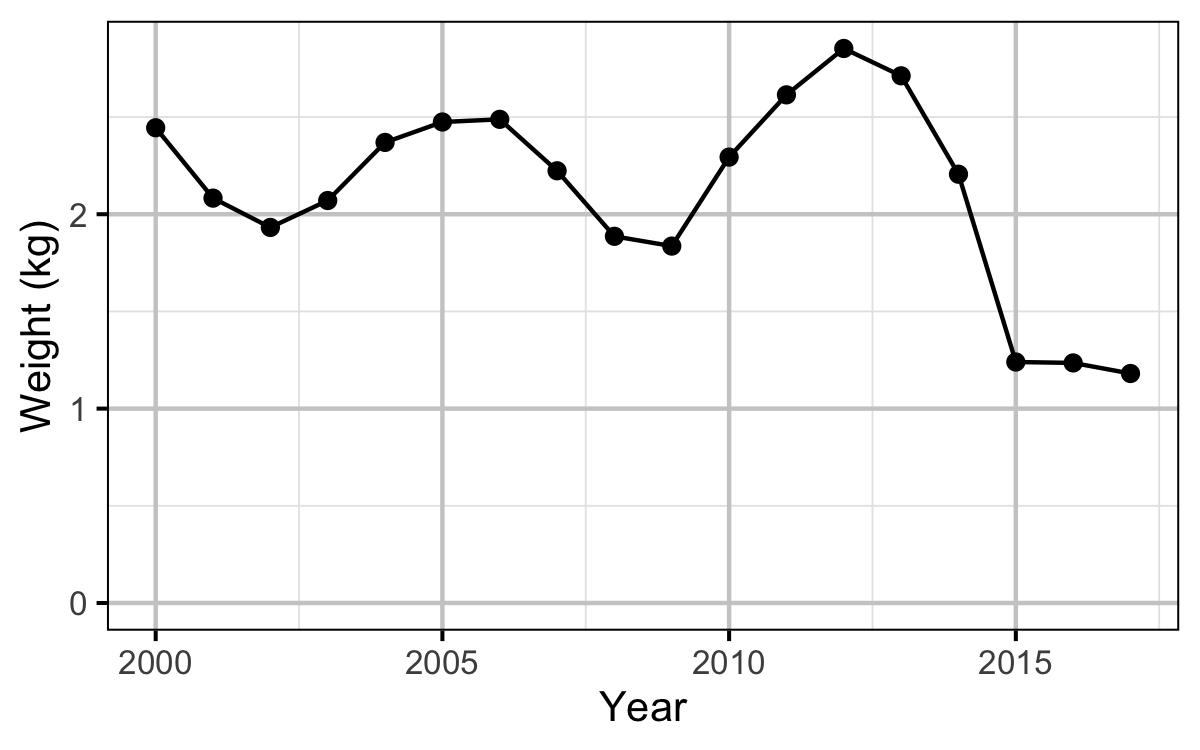Figure 12. The mean weight of Rainbow Trout in the KLRT by year.

#### Stock-Recruitment

##### Age-1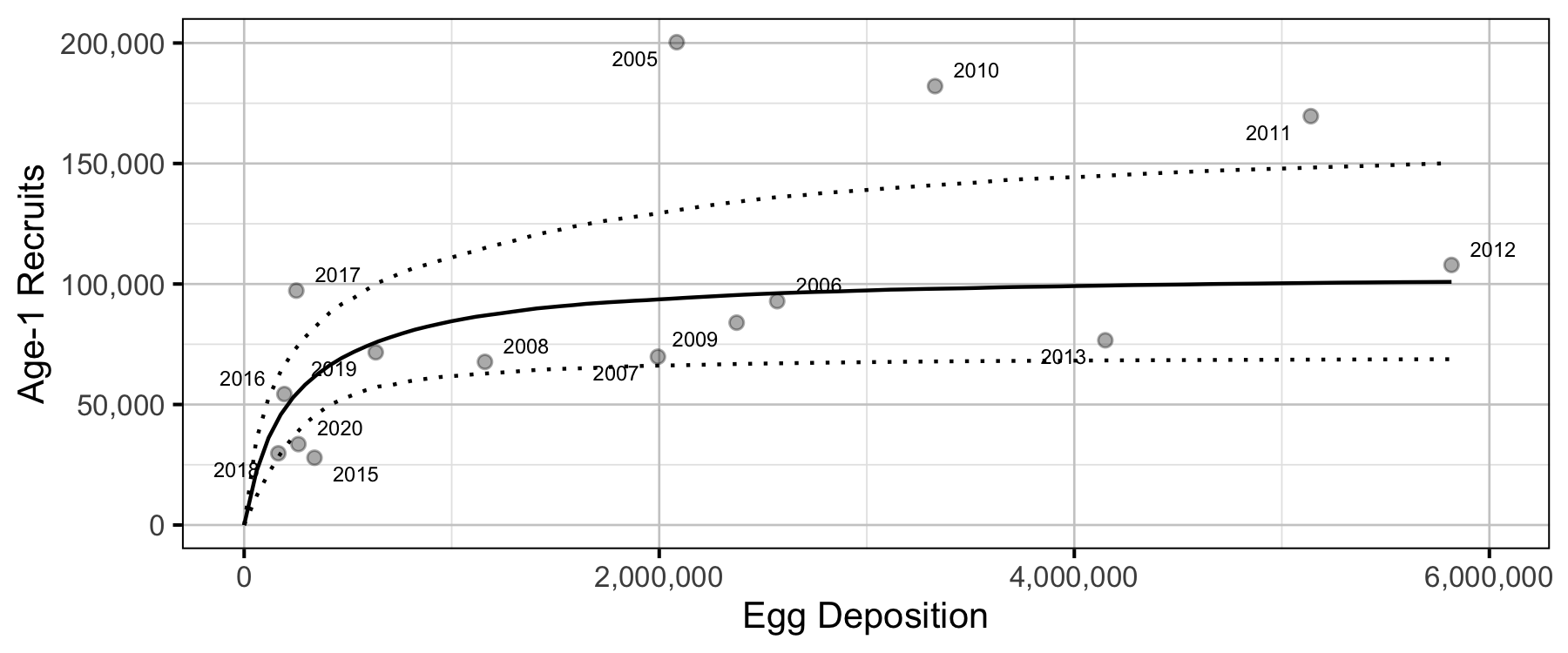Figure 16. Predicted stock-recruitment relationship between spawners and age-1 recruits (with 95% CRIs).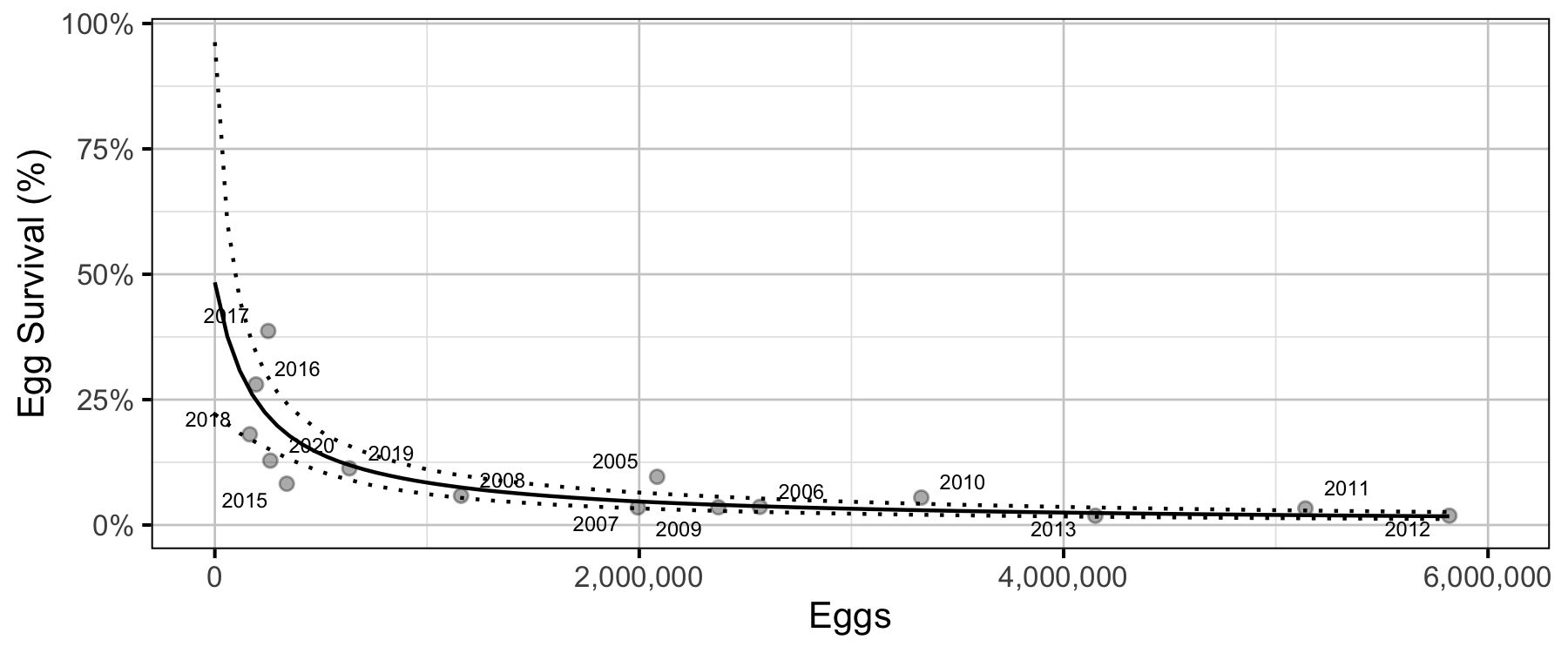Figure 17. Predicted egg survival to age-1 by egg deposition (with 95% CRIs).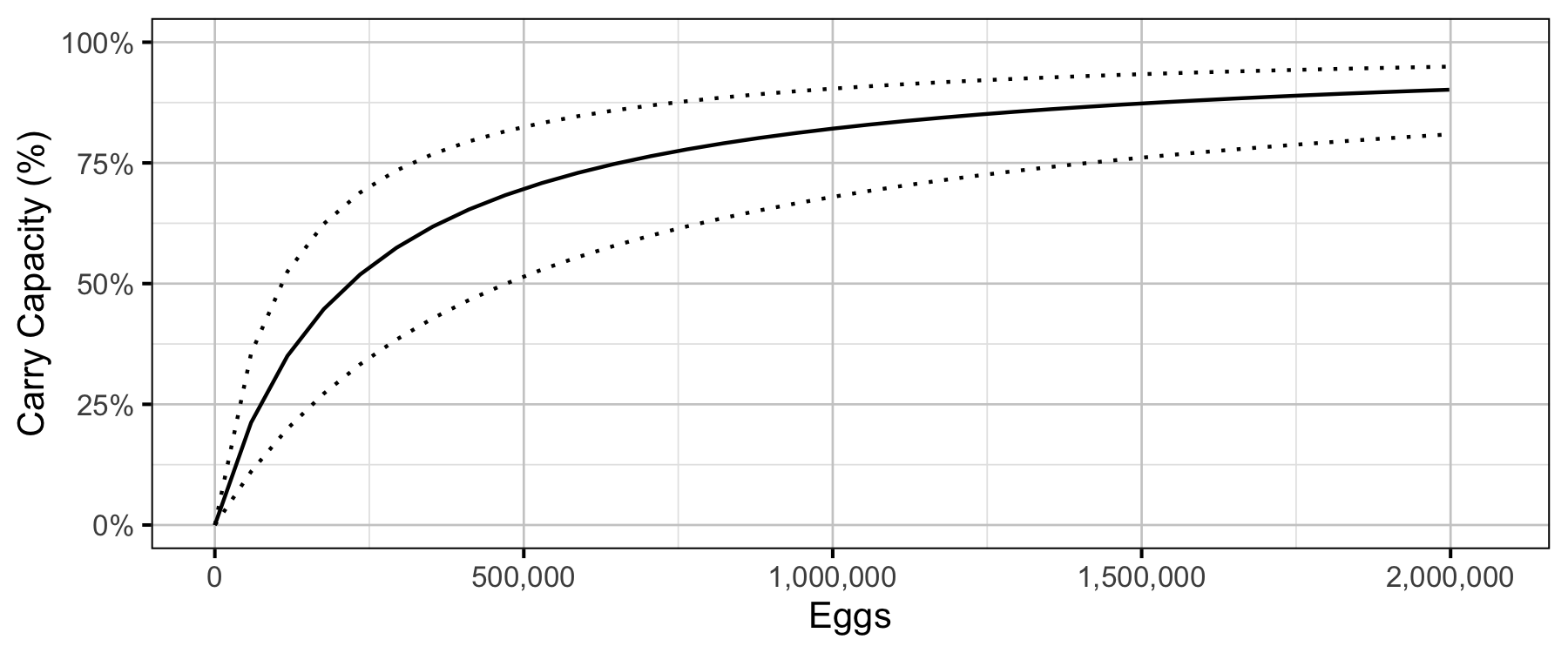Figure 18. Predicted percent of age-1 recruits carry capacity by egg deposition (with 80% CRIs).

#### Age-1 to Age-2 Survival

##### Age-2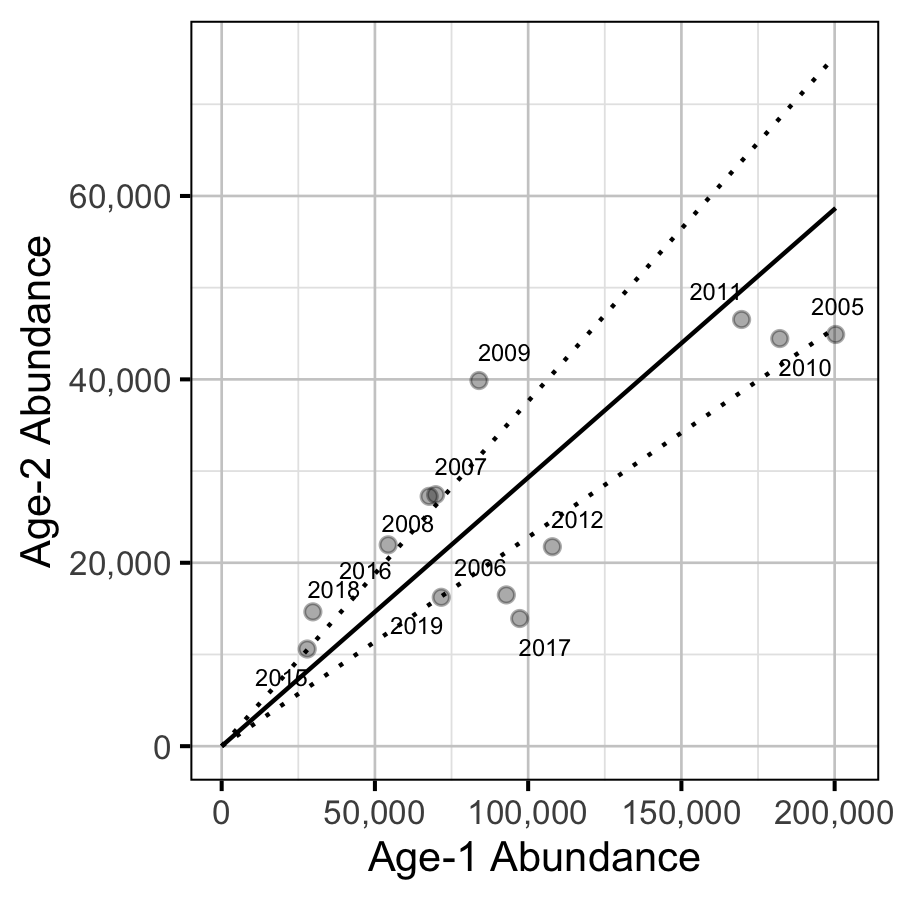Figure 19. Predicted relationship between age-1 and age-2 abundance (with 95% CRIs).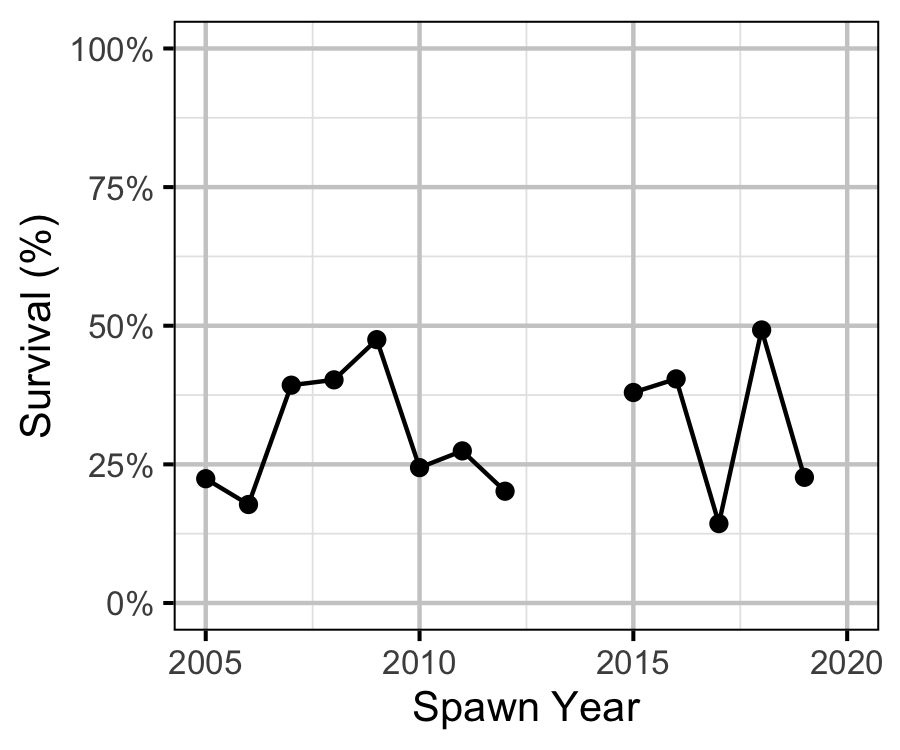Figure 20. Estimated age-1 and age-2 survival by spawn year.

#### Inlake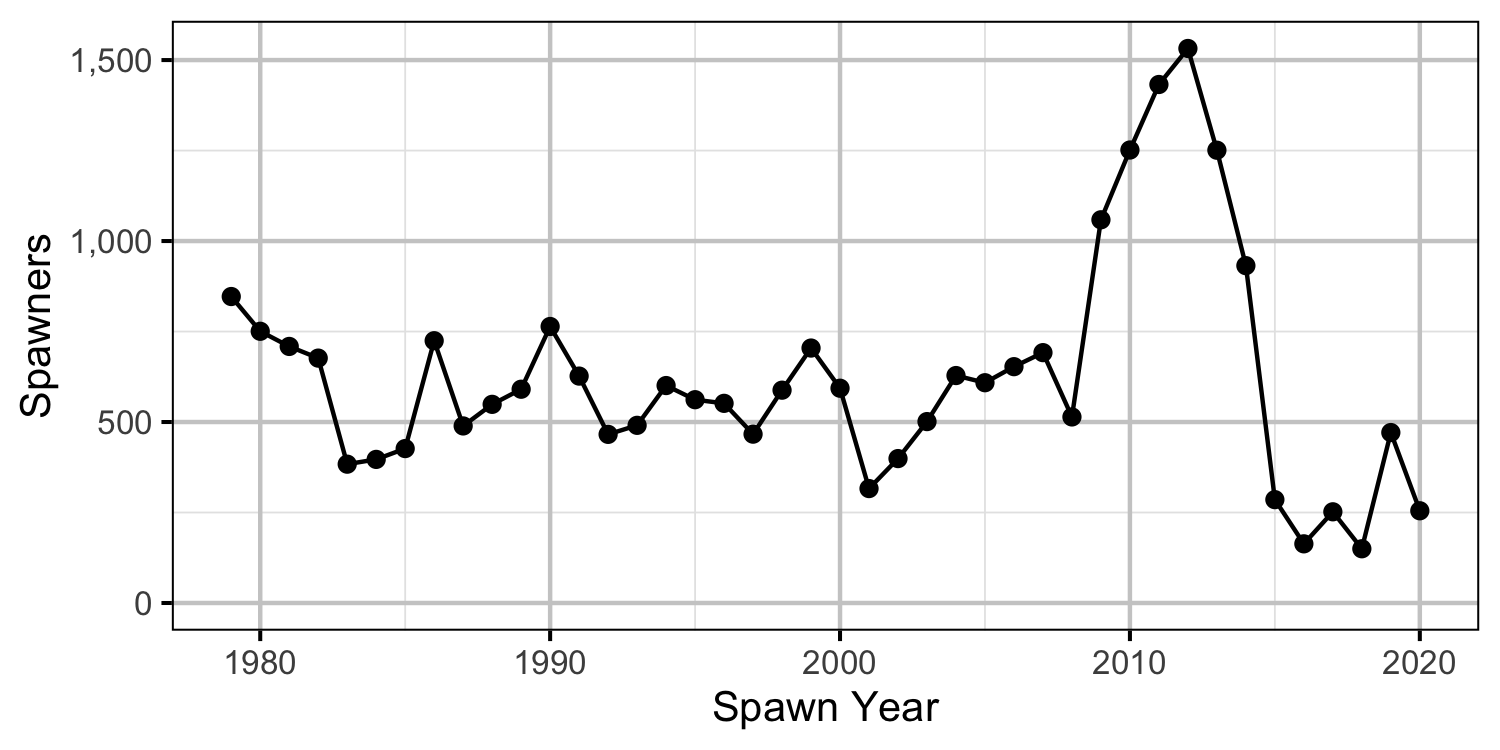Figure 21. The number of spawners by return year.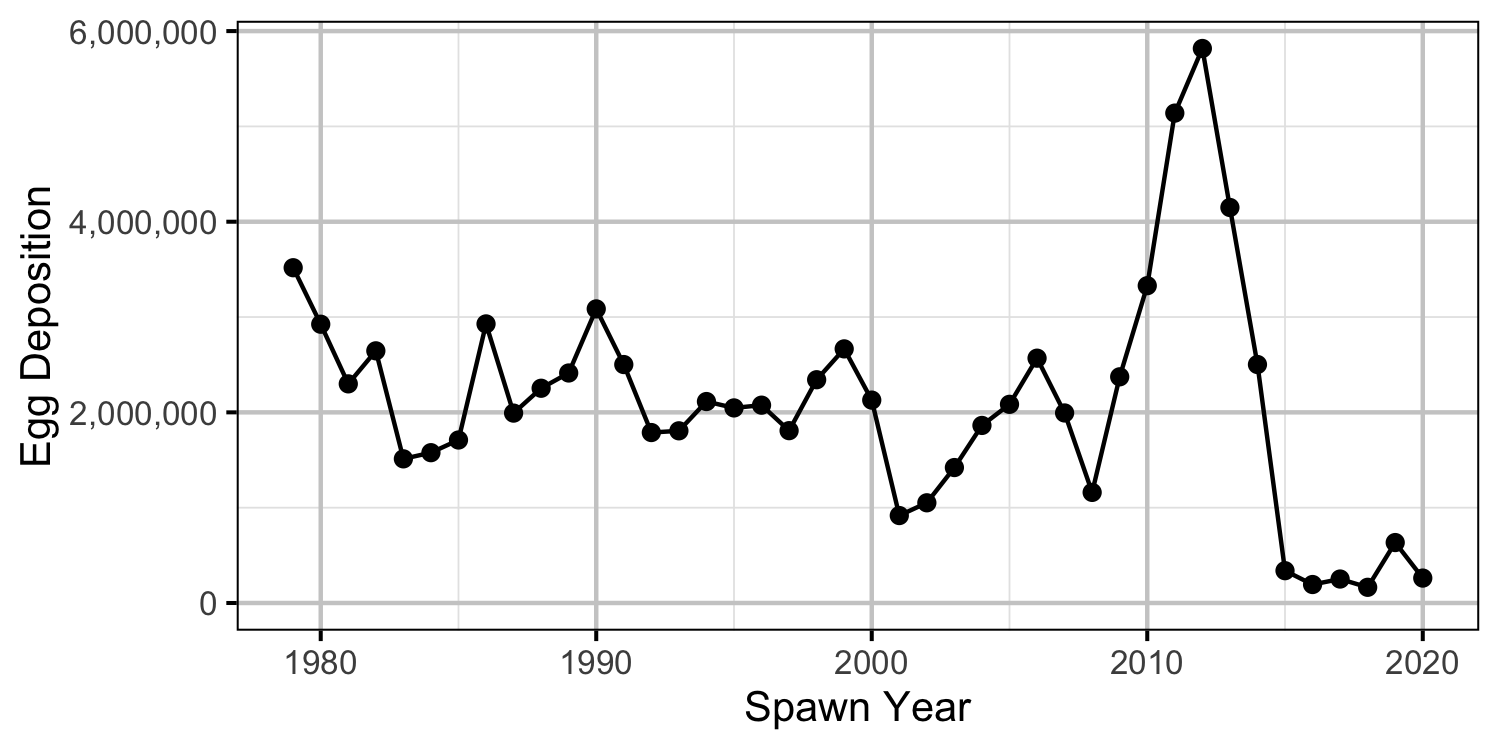Figure 22. The estimated egg deposition by return year.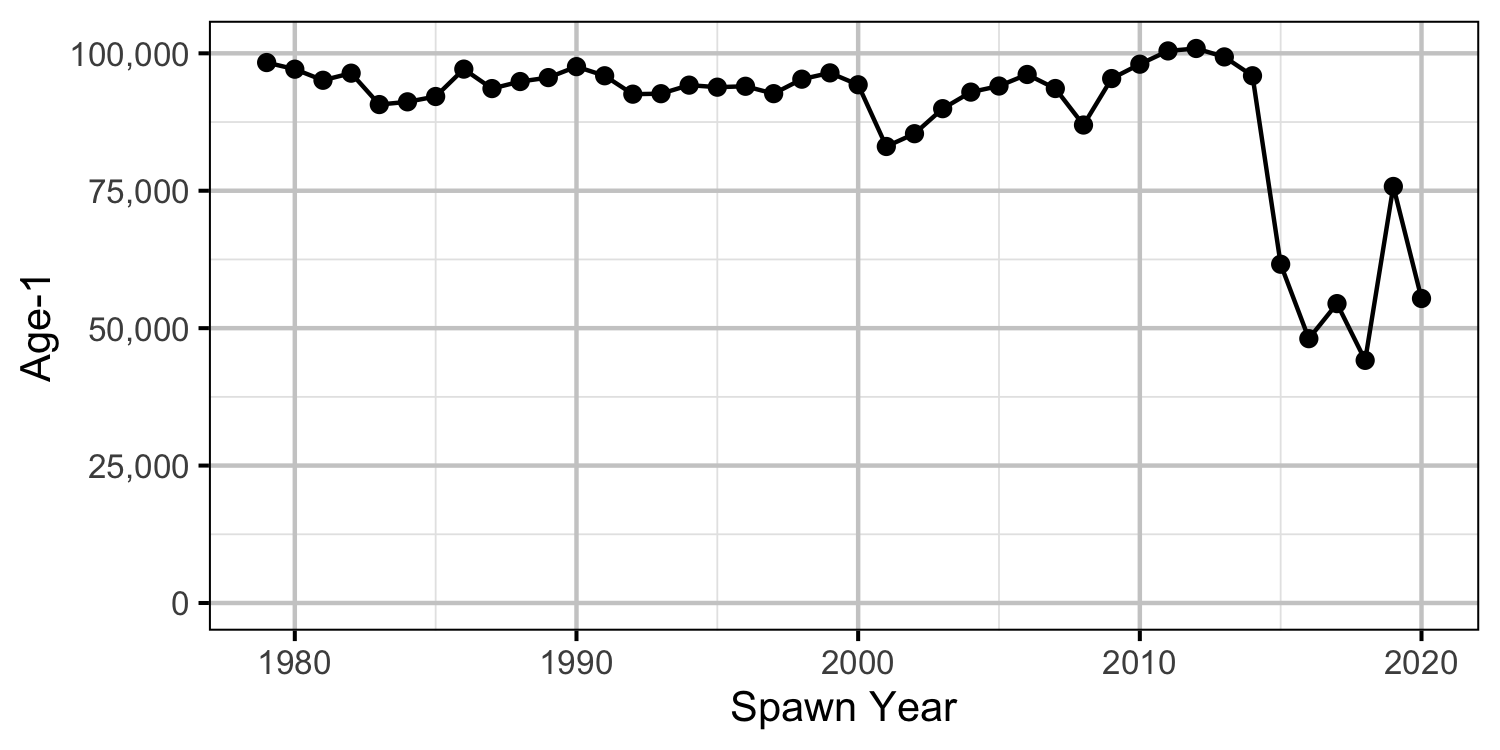Figure 23. The number of expected age-1 recruits by spawn year based on the estimated egg deposition.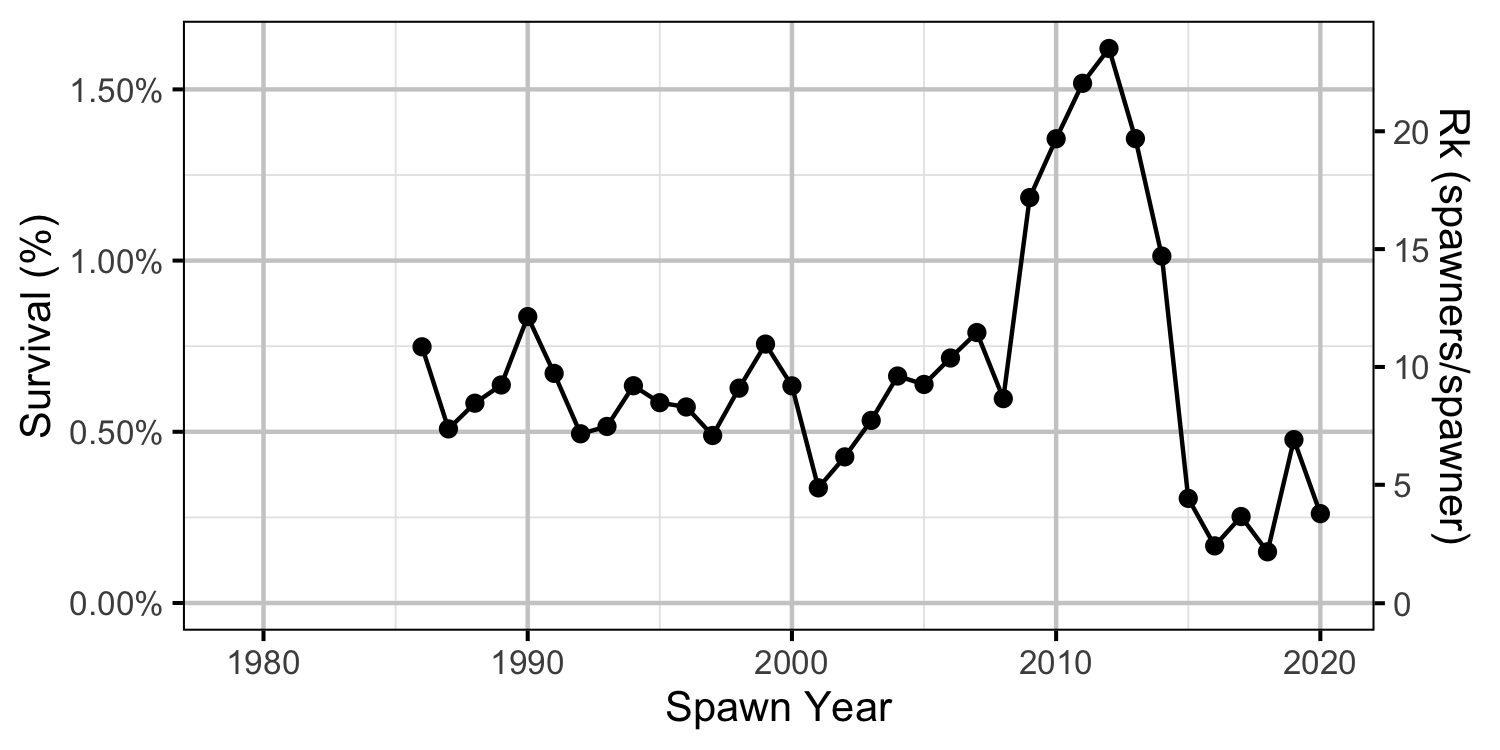Figure 24. Survival from expected age-1 to spawners by return year.